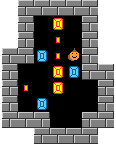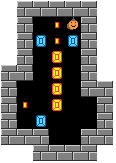Theoretical analysis on Picokosmos 17

by David HollandPicokosmos17

Recurrence of optimal solution variations for a class of puzzles with unusually lengthy solutions

The class of puzzles is based on some puzzle variants first examined by Aymeric du Peloux. These are variants of his puzzle picokosmos#17 with differing numbers of goals. The smallest such puzzle has four goals and looks like this:

```pic17v4g4```

I gave it this name because it was the fourth variant of the picokosmos#17 puzzle I looked at, which seemed to have the longest solution: 19 pushes and 54 steps, or (19,54) for short. The last part of the name (g4) indicates how many goals it has. To get other puzzles in the same class, we "stretch" the rectangle containing the man upwards. So the next puzzle is

```pic17v4g5```

And we carry on in the same way to get other puzzles in the class. Notice here that I have placed the man above the first object to be pushed in the solution, and this alternates between the left and the right depending on whether there are an even or an odd number of goals.

So why is this puzzle (or rather, class of puzzles) so interesting?

First, each member has one of (if not the) longest solutions of any puzzle of its size and number of goals. Note that the start positions of the puzzles have been chosen to be as deep as is possible, based on pushes to reach the solved position. So there can be no different choice of the start position, leaving the goals fixed, which leads to more pushes in a solution. This can be verified directly using computer programs for the first few puzzles in the sequence. In addition, for the smallest puzzle in the sequence, though I haven't tried every way to rearrange the four goals, the several I did try gave solutions at best no longer than for this one.

Second, what links the puzzles in the class is that their solutions are related by a kind of equivalence relation. If we let P(n) be the number of pushes in a push-minimal solution of pic17v4gn (for any number of goals n at least 4) then we have the second order recurrence relation:

P(n) = P(n-1) + P(n-2) +7 for all n >= 4.

This double summing of the pushes makes the solution lengths reach incredible numbers rapidly. Though P(4) is "only" 19, P(5) is 35, which by the relation means

P(6) = 19 + 35 + 7 = 61, P(7) = 61 + 35 + 7 = 103, P(8) = 103 + 61 + 7 = 171, P(9) = 171 + 103 + 7 = 281, P(10) = 281 + 171 + 7 = 459, P(11) = 459 + 281 + 7 = 747, P(12) = 747 + 459 + 7 = 1213.

Thus we find that the pic17g4g12 requires well over a thousand pushes to solve!

What about steps (or man moves)?

It turns out that for this class of puzzles, it is possible to minimise both steps and pushes at the same time, so getting a truly optimal solution. Moreover, for the smaller puzzles this is again possible to do directly using a computer program. Perhaps surprisingly, the optimal solutions which are produced this way fit into a kind of "recurrence relation" of Sokoban moves. Namely, to get the solution to pic17v4gn, we essentially combine the solutions to the two previous puzzles in the series, along with a few extra moves to link them, as might be suggested by the equivalence relation for pushes. As a result, there is another such relation for steps, though it is a little more complex. If S(n) is the number of steps in an optimal solution for pic17v4gn, then

S(n) = S(n-1) + S(n-2) + n + 15, for all n even at least 6,

S(n) = S(n-1) + S(n-2) + n + 17, for all n odd at least 7.

Thus, the number of steps, or man moves, reaches even more dizzying heights rapidly with increasing numbers of goals. For example S(8) is 500.

It is quite straightforward to see how this recurrence of solutions works. We can illustrate with the examples for n=6 for even numbers of goals, and n=7 for odd numbers of goals.

Here is an optimal solution for n=6 as a universal bookmark:

```pic17v4g6 DDDRUldlddRRUrrdLdlUlluurDuurrDDLUdddrUruLulllddRRUrrdLdlUlluurDuuuurrDDLU
rdDDLUdddrUruLulllddRRUdlluurDrddrUruLuuullDDRUldlddRRUrrdLdlUlluurDuurrDD LUdddrUruLulllddRRULrrrdLdlU
```

First we do the first 5 pushes and 7 steps in the solution to reach the position:

```pic17v4g6.5 ```

Notice that the lower part of the puzzle is exactly the same as the position for the four goal puzzle, after the first push down on the left. So we can copy the moves of the four goal puzzle from this point, with the proviso that we stop a couple of pushes before the end (to retain access to both sides of the puzzle):

```pic17v4g6.20 ```

Now we do a specific sequence of seven pushes, which you can follow in the solution given above to reach the position:

```pic17v4g6.27 ```

This sequence generalises to any of the puzzles with an even number of goals: we moved the bottom two objects, and then ran up to the top of the puzzle along the left hand side, and moved the top three objects. The result is that the lower part of the puzzle now looks like the five goal puzzle after the first push, down on the right this time. So we can now copy the solution for the five goal puzzle from this point on, and since the top goal in the six goal puzzle is already stacked, we have finished our solution for the six goal puzzle, and built it up recursively from the four and five goal solutions. Perhaps another example (for 8 goals) would convince, but it should be clear that this works for any even number of goals.

An optimal solution for the 7 goal puzzle is given as a universal bookmark:

```pic17v4g7 DDDLUrdDDLUdddrUruLulllddRRUdlluurDrddrUruLuuullDDRUldlddRRUrrdLdlUlluur
DuurrDDLUdddrUruLulllddRRUdlluurDrddrUruLuuuuullDDRUldDDRUldlddRRUrrdLdl UlluurDuurrDDLUdddrUruLulllddRRUrrdLdlUlluurDuuuurrDDLUrdDDLUdddrUruLull
lddRRUdlluurDrddrUruLuuullDDRUldlddRRUrrdLdlUlluurDuurrDDLUdddrUruLullld dRRULrrrdLdlU```

To build this up recursively, we follow the same basic pattern as for the six goal puzzle above, with a few simple changes. First, we do the mirror image of the first 5 pushes since the man is on the other side. After this we copy the solution for the five goal puzzle, after one push, except for the last two pushes. Then we do a slightly different sequence of 7 pushes to link the puzzles, since the original puzzle is not symmetric, which you can follow in the solution given above. Again we move the bottom two objects, then move back around the right hand side of the puzzle this time, and move the top three objects. We can then copy the solution for the six goal puzzle, after the first push, to complete things.

Summary and future problems

What we have actually shown is that there exists a solution for the stated puzzle class for any number of goals at least 4, and that the solutions are linked recursively in the manner explained above (with various recurrence relations for pushes and steps as a result). With a computer we can determine for the first few puzzles in the class that the start positions are deepest possible, in number of pushes, and that the solutions given are optimal, both for pushes and steps. But at some point it takes too long or too much memory for a computer to determine this information for larger puzzles in the class. So we don't actually know, though it seems likely, that the solutions for larger puzzles we have given are optimal, nor that the start positions are deepest possible in pushes. For instance, on my computer I can determine all this information up to about 8 goals in reasonable time constraints.

In theory, it ought to be possible to program a computer to generate the solution to any puzzle in this class, by putting together the known solutions for the smaller puzzles recursively, and building up through 4,5,6, goals etc. This is a very efficient process and should enable the recursive solutions for very large numbers of goals (say a hundred, perhaps a thousand) to be computed. But with such large puzzles we would then have the anomaly of not being able to check directly that such solutions are optimal - assuming that no proof is forthcoming for the optimality of all such solutions.

For the first puzzle in the class, it might be possible to generate all puzzles of its size (19 squares) with four goals, and determine directly with a computer that indeed it has the longest possible solution (or if not, to find another puzzle that has a longer solution). But for larger puzzles, from some number of goals, this wold be an open question as there are too many possible puzzles to search through. But there is room for guesswork, and the challenge of attempting to beat some puzzle in the class for length of solution.

Finally, note for the mathematically curious that the recurrence relation for pushes, apart from the constant term, is the same as the Fibonacci sequence of integers 1,1,2,3,5,8, etc. I don't know if this is at all significant. Another challenge might be to find a class of puzzles whose solutions (if recursive, or known some other way) rise faster than the given recurrence relation.

Some more analysis from Yaron Shoham

I want to add that the recursions can be transformed to the exact Fibonacci recursion, and then can be solved explicitly.

The number of pushes, P(n), satisfies:

```* P(n) = P(n-1) + P(n-2) + 7
define:X(n) = P(n) + 7
Then, by substitution: X(n) = X(n-1) + X(n-2)```

i.e. X(n) satisfies the Fibonacci recursion. Furthermore, since P(4),P(5),P(6) = 19,35,61,... X(4),X(5),X(6) = 26,42,68,... = 2 * 13,21,34,... it follows that X(n)/2 is part of the original Fibonacci sequence.

The Fibbonaci sequence can be generated by: * F(n) = r*(a^n) - r*(b^n) where a,b are the roots of x^2 - x - 1, and r=1/sqrt(5).

```a = (1 + sqrt(5)) / 2
b = (1 - sqrt(5)) / 2
(I will not prove it here).```

Therefore, generating X(n) and P(n) is simple.

David's analysis also defines S(n), the number of steps.

```* S(n) = S(n-1) + S(n-2) + n + 15, for all n even at least 6,
* S(n) = S(n-1) + S(n-2) + n + 17, for all n odd at least 7.
where S(4) = 54 , S(5) = 101 , S(6) = 176 ...

In short, * S(n) = S(n-1) + S(n-2) + n + 16 + (-1)^(n+1) ```

The transformation here is a bit trickier.

```define: Y(n) = S(n) + n + 19 - (-1)^(n+1)
Then: Y(n) = Y(n-1) + Y(n-2)
because:
Y(n) = S(n) +
n + 19 - (-1)^(n+1) =

S(n-1) +
S(n-2) +
n + 16 + (-1)^(n+1) +
n + 19 - (-1)^(n+1) =

Y(n-1) - (n-1) - 19 + (-1)^(n) +
Y(n-2) - (n-2) - 19 + (-1)^(n-1) +
n + 16 + (-1)^(n+1) +
n + 19 - (-1)^(n+1) =

Y(n-1) + Y(n-2)
```

So Y(n) satisfies the Fibonacci recursion, but it is not the original sequence: Y(4) = 78 , Y(5) = 124, Y(6) = 202, Y(7) = 326 ...

However, Y(n) can be generated in a similar way by choosing:

```c = 9 + sqrt(5)
d = 9 - sqrt(5)

Then
Y(n) = c*(a^n) + d*(b^n)
```

ACCUEIL / WELCOME !